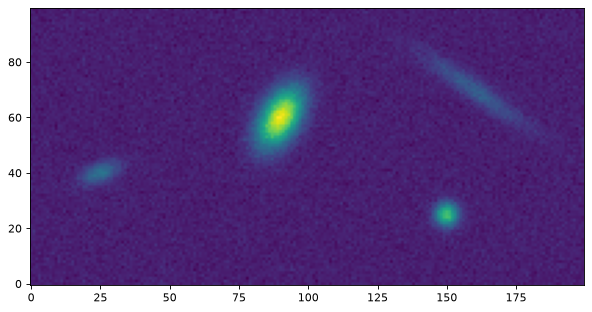# Centroids (photutils.centroids)¶

## Introduction¶

photutils.centroids provides several functions to calculate the centroid of a single source. The centroid methods are:

Masks can be input into each of these functions to mask bad pixels. Error arrays can be input into the two fitting methods to weight the fits.

## Getting Started¶

Let’s extract a single object from a synthetic dataset and find its centroid with each of these methods. For this simple example we will not subtract the background from the data (but in practice, one should subtract the background):

>>> from photutils.datasets import make_4gaussians_image
>>> from photutils import centroid_com, centroid_1dg, centroid_2dg
>>> data = make_4gaussians_image()[43:79, 76:104]

>>> x1, y1 = centroid_com(data)
>>> print((x1, y1))
(13.93157998341213, 17.051234441067088)

>>> x2, y2 = centroid_1dg(data)
>>> print((x2, y2))
(14.040352707371396, 16.962306463644801)

>>> x3, y3 = centroid_2dg(data)
>>> print((x3, y3))
(14.002212073733611, 16.996134592982017)


Now let’s plot the results. Because the centroids are all very similar, we also include an inset plot zoomed in near the centroid:

from photutils.datasets import make_4gaussians_image
from photutils import centroid_com, centroid_1dg, centroid_2dg
import matplotlib.pyplot as plt

data = make_4gaussians_image()[43:79, 76:104]  # extract single object
x1, y1 = centroid_com(data)
x2, y2 = centroid_1dg(data)
x3, y3 = centroid_2dg(data)
fig, ax = plt.subplots(1, 1)
ax.imshow(data, origin='lower', interpolation='nearest', cmap='viridis')
marker = '+'
ms, mew = 30, 2.
plt.plot(x1, y1, color='#1f77b4', marker=marker, ms=ms, mew=mew)
plt.plot(x2, y2, color='#17becf', marker=marker, ms=ms, mew=mew)
plt.plot(x3, y3, color='#d62728', marker=marker, ms=ms, mew=mew)

from mpl_toolkits.axes_grid1.inset_locator import zoomed_inset_axes
from mpl_toolkits.axes_grid1.inset_locator import mark_inset
ax2 = zoomed_inset_axes(ax, zoom=6, loc=9)
ax2.imshow(data, interpolation='nearest', origin='lower',
cmap='viridis', vmin=190, vmax=220)
ax2.plot(x1, y1, color='#1f77b4', marker=marker, ms=ms, mew=mew)
ax2.plot(x2, y2, color='#17becf', marker=marker, ms=ms, mew=mew)
ax2.plot(x3, y3, color='#d62728', marker=marker, ms=ms, mew=mew)
ax2.set_xlim(13, 15)
ax2.set_ylim(16, 18)
mark_inset(ax, ax2, loc1=3, loc2=4, fc='none', ec='0.5')
ax2.axes.get_xaxis().set_visible(False)
ax2.axes.get_yaxis().set_visible(False)
ax.set_xlim(0, data.shape-1)
ax.set_ylim(0, data.shape-1)## Reference/API¶

This subpackage contains tools for centroiding sources.

### Functions¶

 centroid_1dg(data[, error, mask]) Calculate the centroid of a 2D array by fitting 1D Gaussians to the marginal x and y distributions of the array. centroid_2dg(data[, error, mask]) Calculate the centroid of a 2D array by fitting a 2D Gaussian (plus a constant) to the array. centroid_com(data[, mask, oversampling]) Calculate the centroid of an n-dimensional array as its “center of mass” determined from moments. centroid_epsf(data[, mask, oversampling, …]) Calculates centering shift of data using pixel symmetry, as described by Anderson and King (2000; PASP 112, 1360) in their ePSF-fitting algorithm. centroid_sources(data, xpos, ypos[, …]) Calculate the centroid of sources at the defined positions. fit_2dgaussian(data[, error, mask]) Fit a 2D Gaussian plus a constant to a 2D image. gaussian1d_moments(data[, mask]) Estimate 1D Gaussian parameters from the moments of 1D data.

### Classes¶

 GaussianConst2D([constant, amplitude, …]) A model for a 2D Gaussian plus a constant.